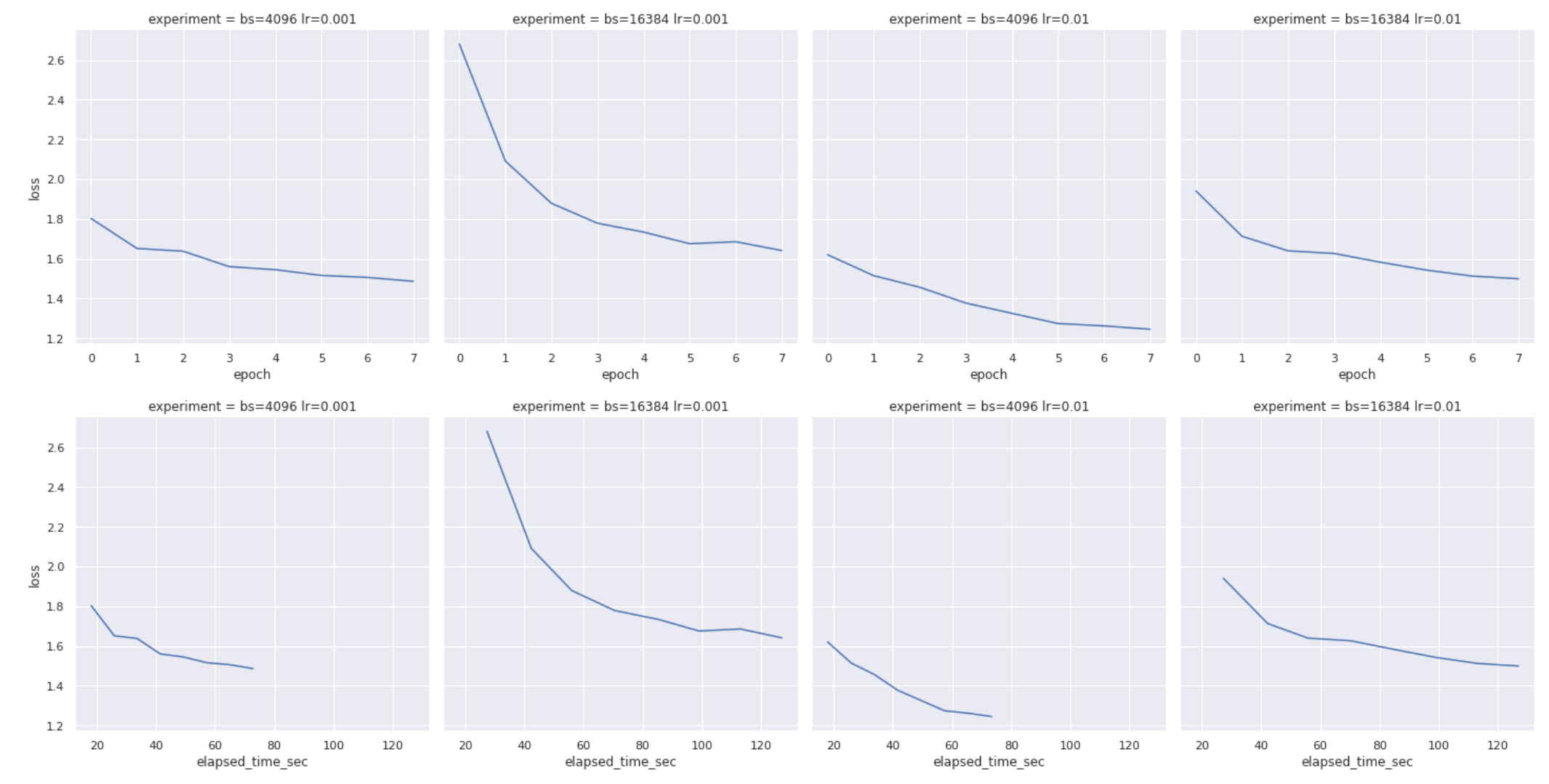# Training many PyTorch models Concurrently with Dask

Train multiple models with at the same time with Dask

This example builds on the getting started with PyTorch example that trains a neural network to generate pet names, but instead of training the model once it trains it several times with different parameters and compares the results. Rather than having to wait for each of the different set of parameters to train sequentially, it uses the Dask cluster to train them all concurrently, dramatically speeding up the process.

all the set up code is the exact same as the original getting started with PyTorch example:

``````import uuid  # noqa
import datetime
import pickle  # noqa
import json
import torch
import torch.nn as nn
import torch.optim as optim
import numpy as np  # noqa
import urllib.request
import pandas as pd
``````
``````with urllib.request.urlopen(
) as f:

# Our list of characters, where * represents blank and + represents stop
characters = list("*+abcdefghijklmnopqrstuvwxyz-. ")
str_len = 8

def format_training_data(pet_names, device=None):
def get_substrings(in_str):
# add the stop character to the end of the name, then generate all the partial names
in_str = in_str + "+"
res = [in_str[0:j] for j in range(1, len(in_str) + 1)]
return res

pet_names_expanded = [get_substrings(name) for name in pet_names]
pet_names_expanded = [item for sublist in pet_names_expanded for item in sublist]
pet_names_characters = [list(name) for name in pet_names_expanded]
pet_names_padded = [name[-(str_len + 1) :] for name in pet_names_characters]
list((str_len + 1 - len(characters)) * "*") + characters for characters in pet_names_padded
]
pet_names_numeric = [[characters.index(char) for char in name] for name in pet_names_padded]

# the final x and y data to use for training the model. Note that the x data needs to be one-hot encoded
if device is None:
y = torch.tensor([name[1:] for name in pet_names_numeric])
x = torch.tensor([name[:-1] for name in pet_names_numeric])
else:
y = torch.tensor([name[1:] for name in pet_names_numeric], device=device)
x = torch.tensor([name[:-1] for name in pet_names_numeric], device=device)
x = torch.nn.functional.one_hot(x, num_classes=len(characters)).float()
return x, y

class OurDataset(Dataset):
def __init__(self, pet_names, device=None):
self.x, self.y = format_training_data(pet_names, device)
self.permute()

def __getitem__(self, idx):
idx = self.permutation[idx]
return self.x[idx], self.y[idx]

def __len__(self):
return len(self.x)

def permute(self):
self.permutation = torch.randperm(len(self.x))

class Model(nn.Module):
def __init__(self):
super(Model, self).__init__()
self.lstm_size = 128
self.lstm = nn.LSTM(
input_size=len(characters),
hidden_size=self.lstm_size,
num_layers=4,
batch_first=True,
dropout=0.1,
)
self.fc = nn.Linear(self.lstm_size, len(characters))

def forward(self, x):
output, state = self.lstm(x)
logits = self.fc(output)
return logits
``````

## Training multiple models in parallel

Below is the code to train the model multiple times concurrently in a distributed way using Dask. Before running the code, check that you’ve started the Dask cluster in your Saturn Cloud Project.

First, we set up the Dask cluster and appropriate libraries.

``````import seaborn as sns
from distributed.worker import logger

n_workers = 3
cluster = SaturnCluster(n_workers=n_workers)
client = Client(cluster)
client.wait_for_workers(n_workers)
``````

The code to run the model multiple times in parallel starts with a training function that is extremely similar to the original one. The changes are:

• The function has a @dask.delayed indicator at the top so Dask knows to parallelize it
• The training function now has an input parameter `experiment` which is a tuple containing the batch size and learning rate
• Instead of printing the results, we now pass them to `logger.info()` so that they show up in the Dask logs.
• Instead of saving the actual model, we create an array of results that the model returns
``````@dask.delayed
def train(experiment):
num_epochs = 8
batch_size, lr = experiment
training_start_time = datetime.datetime.now()
device = torch.device(0)

dataset = OurDataset(pet_names, device=device)

model = Model()
model = model.to(device)

criterion = nn.CrossEntropyLoss()

results = []

for epoch in range(num_epochs):
dataset.permute()
for i, (batch_x, batch_y) in enumerate(loader):
batch_y_pred = model(batch_x)

loss = criterion(batch_y_pred.transpose(1, 2), batch_y)
loss.backward()
optimizer.step()
logger.info(
f"{datetime.datetime.now().isoformat()} - batch {i} - batch_size {batch_size} - lr {lr} - epoch {epoch} complete - loss {loss.item()}"
)
new_results = {
"batch_size": batch_size,
"lr": lr,
"epoch": epoch,
"loss": loss.item(),
"elapsed_time_sec": (datetime.datetime.now() - training_start_time).total_seconds(),
}
results.append(new_results)
return results
``````

### Running the parallel code

The code is executed across multiple machines by running the cell below. It takes the list of (batch size, learning rate) tuples and passes them to the Dask map function, that get combined into a single result with gather and compute. Since Dask is lazy, the computation doesn’t actually begin until you run the last line of the cell and request the results. You won’t see any messages show up as the model is being trained since all of the output is captured in the Dask logs.

``````inputs = [(4096, 0.001), (16384, 0.001), (4096, 0.01), (16384, 0.01)]

train_future = client.map(train, inputs)
futures_gathered = client.gather(train_future)
futures_computed = client.compute(futures_gathered)
results = [x.result() for x in futures_computed]
``````

This last code generates several Seaborn plots of the results of the experiment. With this particular experiment the smallest batch size with highest learning rate quickly convered on a solution with fewer epochs than the other sets of parameters.

``````results_concatenated = [item for sublist in results for item in sublist]
results_df = pd.DataFrame.from_dict(results_concatenated)
results_df["experiment"] = (
"bs=" + results_df["batch_size"].astype(str) + " lr=" + results_df["lr"].astype(str)
)

sns.set_theme()

sns.relplot(data=results_df, x="epoch", y="loss", col="experiment", kind="line")

sns.relplot(data=results_df, x="elapsed_time_sec", y="loss", col="experiment", kind="line")
``````